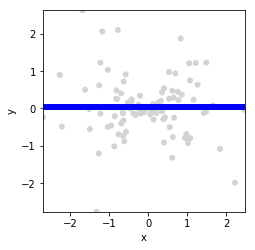# Hline¶

Title
HLine Element
Dependencies
Matplotlib
Backends
Matplotlib
Bokeh
In :
import numpy as np
import holoviews as hv
from holoviews import opts

hv.extension('matplotlib')The HLine element is a type of annotation that marks a position along the y-axis. Here is an HLine element that marks the mean of a points distributions:

In :
xs = np.random.normal(size=100)
ys = np.random.normal(size=100) * xs
points = hv.Points((xs,ys))

(points * hv.HLine(ys.mean())).opts(
opts.HLine(color='blue', linewidth=6),
opts.Points(color='#D3D3D3'))

Out:For full documentation and the available style and plot options, use hv.help(hv.HLine).